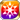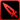# A Taste of Bliss at the Promised Time

Type
Equipment
Rank
5★
Max level
40
Enhancer cost
2000
Enhancer EXP
20000
Vision Clear Reward
Source
Event (Promises Under the Harvest Moon)
Release dates
• JP: 2019-09-19
• GL: 2020-08-20
AlchemistCodeDBIt was so wonderful, it's hard to believe that I was actually there. Tamamo was in a world of bliss as she enjoyed the dumplings we made, exclaiming, "Mikoto! We finally got to do it! Moon-gazing is the best!" There are those who are frightened of her and some might say she's too selfish, but she's an important friend of mine. She never forgot the promise we made to each other all that time ago, and even went so far as to put this moon-gazing event together for my sake. I cannot help but want to pray for her happiness, and I hope that the Gods will favor her. (Mikoto's Outings Diary)

## Stats

#### Simple stats

TypeLvl 1Lvl 30Lvl 40
LUCK+7+16+20

LevelLUCK
1+7
2+7
3+7
4+8
5+8
6+8
7+9
8+9
9+9
10+10
LevelLUCK
11+10
12+10
13+11
14+11
15+11
16+12
17+12
18+12
19+13
20+13
LevelLUCK
21+13
22+14
23+14
24+14
25+15
26+15
27+15
28+16
29+16
30+16
LevelLUCK
31+17
32+17
33+17
34+18
35+18
36+18
37+19
38+19
39+19
40+20

## Group skills

#### Simple stats

TypeLvl 1Lvl 30Lvl 40
MDEF+13+33+40

LevelMDEF
1+13
2+13
3+14
4+15
5+15
6+16
7+17
8+17
9+18
10+19
LevelMDEF
11+19
12+20
13+21
14+22
15+22
16+23
17+24
18+24
19+25
20+26
LevelMDEF
21+26
22+27
23+28
24+28
25+29
26+30
27+31
28+31
29+32
30+33
LevelMDEF
31+33
32+34
33+35
34+35
35+36
36+37
37+37
38+38
39+39
40+40

#### Limit Break

TypeLB1LB2LB3LB4LB5
MDEF+5+9+14+19+24

#### Simple stats

TypeLvl 1Lvl 30Lvl 40
MDEF+13+33+40

LevelMDEF
1+13
2+13
3+14
4+15
5+15
6+16
7+17
8+17
9+18
10+19
LevelMDEF
11+19
12+20
13+21
14+22
15+22
16+23
17+24
18+24
19+25
20+26
LevelMDEF
21+26
22+27
23+28
24+28
25+29
26+30
27+31
28+31
29+32
30+33
LevelMDEF
31+33
32+34
33+35
34+35
35+36
36+37
37+37
38+38
39+39
40+40

#### Limit Break

TypeLB1LB2LB3LB4LB5
MDEF+5+9+14+19+24

### Shrine of Spirits

#### Simple stats

TypeLvl 1Lvl 30Lvl 40
Area ATK Res+2+4+5
Sleep Res+11+26+32
AGI+2+4+6

#### Detailed stats

LevelArea ATK ResSleep ResAGI
1+2+11+2
2+2+11+2
3+2+12+2
4+2+12+2
5+2+13+2
6+2+13+2
7+2+14+2
8+2+14+2
9+2+15+2
10+2+15+2
LevelArea ATK ResSleep ResAGI
11+2+16+3
12+2+16+3
13+2+17+3
14+3+18+3
15+3+18+3
16+3+19+3
17+3+19+3
18+3+20+3
19+3+20+3
20+3+21+3
LevelArea ATK ResSleep ResAGI
21+3+21+4
22+3+22+4
23+3+22+4
24+3+23+4
25+3+23+4
26+3+24+4
27+4+25+4
28+4+25+4
29+4+26+4
30+4+26+4
LevelArea ATK ResSleep ResAGI
31+4+27+5
32+4+27+5
33+4+28+5
34+4+28+5
35+4+29+5
36+4+29+5
37+4+30+5
38+4+30+5
39+4+31+5
40+5+32+6

#### Limit Break

TypeLB1LB2LB3LB4LB5
Area ATK Res+1+1+2+2+3
Sleep Res+4+8+12+16+20
AGI+1+1+2+3+4

### Kuzunoha Power

#### Simple stats

TypeLvl 1Lvl 30Lvl 40
Wind+2+4+6
PATK+15+41+50

LevelWindPATK
1+2+15
2+2+15
3+2+16
4+2+17
5+2+18
6+2+19
7+2+20
8+2+21
9+2+22
10+2+23
LevelWindPATK
11+3+23
12+3+24
13+3+25
14+3+26
15+3+27
16+3+28
17+3+29
18+3+30
19+3+31
20+3+32
LevelWindPATK
21+4+32
22+4+33
23+4+34
24+4+35
25+4+36
26+4+37
27+4+38
28+4+39
29+4+40
30+4+41
LevelWindPATK
31+5+41
32+5+42
33+5+43
34+5+44
35+5+45
36+5+46
37+5+47
38+5+48
39+5+49
40+6+50

#### Limit Break

TypeLB1LB2LB3LB4LB5
Wind+1+1+2+3+4
PATK+6+12+18+24+30

TypeLB5
Buff Duration+1

## Vision abilities

### Limit Reached Bonus

#### Ability base

• Slot:Support

Revealed after Limit Reached. Raises AGI after appearing on map.

#### Ability max

• Slot:Action

Raises AGI after appearing on map.

### Unhappy Rain

#### Ability base

• Slot:Action
• DMG Type:Physical
• ATK Type:Missile

Missile ATK on units within area & lowers all Status Res & Jewels Obtained for 3 turns [Range: 3, Area: Square (3), Height Range: Unlimited]

Skill Effect
TypeMinMax
Attack80%100%
• Charges: 2
• Jewel Cost: 40
• Height: 99
• Select Range: Diamond
• Range: 0-3
• Select Scope: Square
• Scope: 1

#### Ability max

• Slot:Action
• DMG Type:Physical
• ATK Type:Missile

Missile ATK on units within area & lowers all Status Res & Jewels Obtained for 3 turns [Range: 3, Area: Square (3), Height Range: Unlimited]

Skill Effect
TypeMinMax
Attack160%160%
• Charges: 2
• Jewel Cost: 40
• Height: 99
• Select Range: Diamond
• Range: 0-3
• Select Scope: Square
• Scope: 1